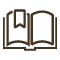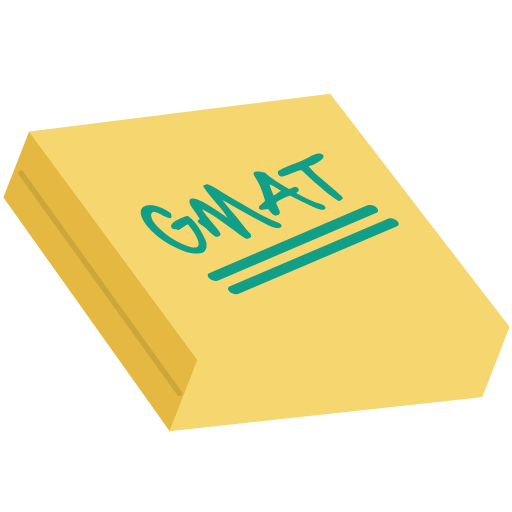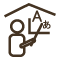# GMAT Quantitative ( MATHS)

25 Mins P 900 Trial 15 Mins P 500SinghEnglishTest PrepGMATHome TutorMath

GMAT Quantitative (or Math) is a 75-minute section where you will get 37 questions.

Regarding Camera Usage

I require both parties to have the camera on. Thank you for your understanding.

GMAT Math Topics

Structure of GMAT Math

GMAT Quantitative (or Math) is 75-minute section where you will get 37 questions. Like Verbal Section, Quant Section is also Computer-Adaptive. You will get harder or easier question, depending on whether your previous answers was correct or wrong. And once you answer a question, you cannot go back to it. There are two main question types:

1.Problem Solving (PS)

2.Data Sufficiency (DS)

Concepts in GMAT Quant .

Arithmetic, Algebra, Geometry, Numbers, Algebraic Expressions, Lines , Real Numbers ,Linear Equations, Angles ,Integers, Quadratic Equations, Triangles, Fractions Exponents ,  Right Triangles ,Decimals,  Quadrilaterals ,Averages ,Absolute Value  Circles, Ratio & Proportion Functions Polygons Percentages

Coordinate Geometry Roots & Powers

Word Problems

•Time Speed (Rate) Problems •Work Problems•Mixture Problems

•Interest Problems•Discount•Profit•Sets and Venn Diagrams

•Geometry Problems•Measurement Problems  •Data Interpretation

Material: Flexible